Next: Probability of complete classes Up: Rules of probability Previous: Rules of probability

Probability of simple propositions

Let us start by recalling the basic rules of probability for propositions or hypotheses. Let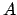andbe propositions, which can take on only two values, for example, true or false. The notationstands for the probability thatis true. The elementary rules of probability for simple propositions are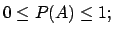(1)(2)(3)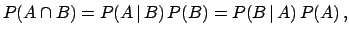(4)

where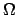means tautology (a proposition that is certainly true). The constructis true only when bothandare true (logical AND), while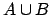is true when at least one of the two propositions is true (logical OR).is also written simply as' or, and is also called a logical product, whileis also called a logical sum.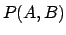is called the joint probability ofand.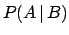is the probability ofunder that condition thatis true. We often read it simply as `the probability of A, given.'' .

Equation (4) shows that the joint probability of two events can be decomposed into conditional probabilities in different two ways. Either of these ways is called the product rule. If the status ofdoes not change the probability of, and the other way around, thenandare said to be independent, probabilistically independent to be precise. In that case,, and, which, when inserted in Eq. (4), yields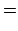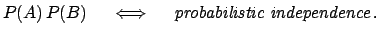(5)

Equations (1)-(4) logically lead to other rules which form the body of probability theory. For example, indicating the negation (or opposite) ofwith, clearly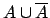is a tautology (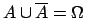), and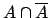is a contradiction (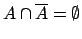). The symbolstands for contradiction (a proposition that is certainly false). Hence, we obtain from Eqs. (2) and (3)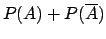(6)

which says that propositionis either true or not true.Next: Probability of complete classes Up: Rules of probability Previous: Rules of probability
Giulio D'Agostini 2003-05-13